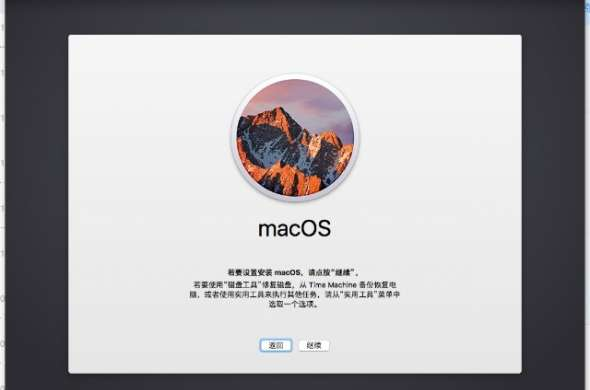•

# 交换语句

## c/c++

```int age;

age = 35;
switch (age) {
case 12:
cout << "你猜错了，福哥没有这么小哦！" << endl;
break;
case 25:
cout << "你猜错了，福哥参加工作很多年了呢！" << endl;
break;
case 35:
cout << "恭喜！猜对了！" << endl;
break;
default:
cout << "这个答案我不是很确定！" << endl;
break;
}```

## java

```String age;

age = "35";
switch (age) {
case "12":
System.out.println("你猜错了，福哥没有这么小哦！");
break;
case "25":
System.out.println("你猜错了，福哥参加工作很多年了呢！");
break;
case "35":
System.out.println("恭喜！猜对了！");
break;
default:
System.out.println("这个答案我不是很确定！");
break;
}```

## php

```\$age = "35";
switch (\$age) {
case "12":
print ("你猜错了，福哥没有这么小哦！\n");
break;
case "25":
print ("你猜错了，福哥参加工作很多年了呢！\n");
break;
case "35":
print ("恭喜！猜对了！\n");
break;
default:
print ("这个答案我不是很确定！\n");
break;
}```

# 交换语句与条件语句

## switch

```int age;
age = 35;
switch (age) {
case 12:
cout << "你猜错了，福哥没有这么小哦！" << endl;
break;
case 25:
cout << "你猜错了，福哥参加工作很多年了呢！" << endl;
break;
case 35:
cout << "恭喜！猜对了！" << endl;
break;
default:
cout << "这个答案我不是很确定！" << endl;
break;
}```

## if

```int age;
age = 35;
if (age == 12) {
cout << "你猜错了，福哥没有这么小哦！" << endl;
}
else if (age == 25) {
cout << "你猜错了，福哥参加工作很多年了呢！" << endl;
}
else if (age == 35) {
cout << "恭喜！猜对了！" << endl;
}
else{
cout << "这个答案我不是很确定！" << endl;
}```

# 总结•• 日志：212
• 回复：13